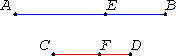# Proposition 19

If a whole is to a whole as a part subtracted is to a part subtracted, then the remainder is also to the remainder as the whole is to the whole.

Let the whole AB be to the whole CD as the part AE subtracted is to the part CF subtracted.

I say that the remainder EB is also to the remainder FD as the whole AB is to the whole CD.V.16

Since AB is to CD as AE is to CF, therefore alternately, BA is to AE as DC is to CF.

And, since the magnitudes are proportional taken jointly, they are also proportional taken separately, that is, BE is to EA as DF is to CF, and, alternately, BE is to DF as EA is to FC.

But, by hypothesis, AE is to CF as is the whole AB to the whole CD.

V.11

Therefore the remainder EB is also to the remainder FD as the whole AB is to the whole CD.

Therefore if a whole is to a whole as a part subtracted is to a part subtracted, then the remainder is also to the remainder as the whole is to the whole.

Q.E.D.

# Corollary

From this it is manifest that, if magnitudes are proportional taken jointly, then they are also proportional in conversion.

## Guide

This proposition says that if (u + v) : (x + y) equals v : y, then it also equals u : x.

The transformations of proportions taken jointly, taken separately, and in conversion are summarized in the Guide for V.Def.14.

The magnitudes in this proposition must all be of the same kind, but those in the corollary can be of two different kinds. Thus, the corollary is out of place. It should probably be after the last proposition since it follows from the previous two propositions by inversion. As Heiberg and Heath agree, the corollary was probably interpolated before Theon’s time.

This proposition relies on using V.Def.4 as an axiom of comparability. (Infinitesimal counterexample: when y is infinitesimal with respect to x, then 2x : (2x + 2y) equals x : (x + 2y) but does not equal x : x.) The corollary, however, does not rely on an axiom of comparability.

#### Use of the proposition and the corollary

This proposition is used in V.25 and a few propositions in Book X. The corollary is used once in each of Books VI and XIII and fairly often in Book X.